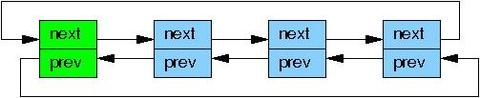What is RCU, Fundamentally?lwn.net1，在读取过程中，另外一个线程删除了一个节点。删除线程可以把这个节点从链表中移除，但它不能直接销毁这个节点，必须等到所有的读取线程读取完成以后，才进行销毁操作。RCU中把这个过程称为宽限期（Grace period）。

2，在读取过程中，另外一个线程插入了一个新节点，而读线程读到了这个节点，那么需要保证读到的这个节点是完整的。这里涉及到了发布-订阅机制（Publish-Subscribe Mechanism）。

3， 保证读取链表的完整性。新增或者删除一个节点，不至于导致遍历一个链表从中间断开。但是RCU并不保证一定能读到新增的节点或者不读到要被删除的节点。

RCU的一个最关键的特性在于，它能够保证数据能安全的被读取(原文用的是scan这个词，我把它翻译成读取貌似比较好？)，即便数据被并发地更新。为了能够并发地插入数据，RCU使用了发布-订阅机制。举个例子，全局指针gp，指向一段新的已分配的空间并初始化，如下：

``````struct foo {
int a;
int b;
int c;
};
struct foo *gp = NULL;

/* . . . */

p = kmalloc(sizeof(*p), GFP_KERNEL);
p->a = 1; // 1
p->b = 2; // 2
p->c = 3; // 3
gp = p;   // 4``````

``````p->a = 1; // 1
p->b = 2; // 2
p->c = 3; // 3
rcu_assign_pointer(gp, p);``````

``````#define rcu_assign_pointer(p, v)					      \
({									      \
uintptr_t _r_a_p__v = (uintptr_t)(v);				      \
\
if (__builtin_constant_p(v) && (_r_a_p__v) == (uintptr_t)NULL)	      \
WRITE_ONCE((p), (typeof(p))(_r_a_p__v));		      \
else								      \
smp_store_release(&p, RCU_INITIALIZER((typeof(p))_r_a_p__v)); \
_r_a_p__v;							      \
})``````

``````p = gp;
if (p != NULL) {
do_something_with(p->a, p->b, p->c);
}``````

``````  rcu_read_lock();
p = rcu_dereference(gp);
if (p != NULL) {
do_something_with(p->a, p->b, p->c);
}

``````#define rcu_dereference(p) rcu_dereference_check(p, 0)

#define rcu_dereference_check(p, c) \

#define __rcu_dereference_check(p, c, space) \
({ \
/* Dependency order vs. p above. */ \
typeof(*p) *________p1 = (typeof(*p) *__force)lockless_dereference(p); \
RCU_LOCKDEP_WARN(!(c), "suspicious rcu_dereference_check() usage"); \
rcu_dereference_sparse(p, space); \
((typeof(*p) __force __kernel *)(________p1)); \
})

#ifdef __CHECKER__
#define rcu_dereference_sparse(p, space) \
((void)(((typeof(*p) space *)p) == p))
#else /* #ifdef __CHECKER__ */
#define rcu_dereference_sparse(p, space)
#endif /* #else #ifdef __CHECKER__ */

#define lockless_dereference(p) \
({ \
typeof(*(p)) *___typecheck_p __maybe_unused; \
smp_read_barrier_depends(); /* Dependency order vs. p above. */ \
(_________p1); \
})``````

RCU中的宽限期

``````struct foo {
int a;
char b;
long c;
};

DEFINE_SPINLOCK(foo_mutex);

struct foo *gbl_foo;

{
foo *fp = gbl_foo; // 如果发生了进程切换
if ( fp != NULL )
dosomething(fp->a, fp->b , fp->c );
}

void foo_update( foo* new_fp )
{
spin_lock(&foo_mutex);
foo *old_fp = gbl_foo;
gbl_foo = new_fp;
spin_unlock(&foo_mutex);
kfee(old_fp);
}``````

``````void foo_update( foo* new_fp )
{
spin_lock(&foo_mutex);
foo *old_fp = gbl_foo;
gbl_foo = new_fp;
spin_unlock(&foo_mutex);
synchronize_rcu();
kfee(old_fp);
}``````

``````static inline void synchronize_rcu(void)
{
synchronize_sched();
}

void synchronize_sched(void)
{
RCU_LOCKDEP_WARN(lock_is_held(&rcu_bh_lock_map) ||
lock_is_held(&rcu_lock_map) ||
lock_is_held(&rcu_sched_lock_map),
"Illegal synchronize_sched() in RCU-sched read-side critical section");
if (rcu_blocking_is_gp())
return;
if (rcu_gp_is_expedited())
synchronize_sched_expedited();
else
wait_rcu_gp(call_rcu_sched);
}

...``````

``````void __wait_rcu_gp(bool checktiny, int n, call_rcu_func_t *crcu_array,
struct rcu_synchronize *rs_array)
{
int i;

/* Initialize and register callbacks for each flavor specified. */
for (i = 0; i < n; i++) {
if (checktiny &&
(crcu_array[i] == call_rcu ||
crcu_array[i] == call_rcu_bh)) {
might_sleep();
continue;
}
init_completion(&rs_array[i].completion);
}

/* Wait for all callbacks to be invoked. */
for (i = 0; i < n; i++) {
if (checktiny &&
(crcu_array[i] == call_rcu ||
crcu_array[i] == call_rcu_bh))
continue;
wait_for_completion(&rs_array[i].completion);
}
}``````

RCU的数据完整性保证

1. 数据插入时，读取数据的保证，考虑下面的情况：

2. 数据删除时，读取数据的保证，考虑下面的情况：

3. 数据更新时，读取数据的保证，考虑下面的情况：